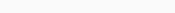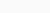# SSC CHSL Practice Paper

### Section 2: Reasoning : SSC CHSL Practice Paper

Q.1: Select the word-pair in which the two words are related in the same way as are the two words in the following word-pair.
Language : Telugu
(A) Hindi : India
(B) Vehicle : Mechanic
(C) Continent : Asia
(D) Society : Service

Ans : (C) Continent : Asia

Q.2: Select the combination of letters that when sequentially placed in the blanks of the given letter series will complete the series.
Z H _ O R C _ _ K _ R _ Z H _ o _ C
(A) Z,H,K,O,C,K,R
(B) K,Z,H,K,R,K,R
(C) K,Z,H,O,C,K,R
(D) K,Z,O,R,C,K,R

Ans : (C) K,Z,H,O,C,K,R

Q.3: How many triangles are there in the figure given below?

(A) 8
(B) 10
(C) 9
(D) 11

Ans : (B) 10

Q.4: In a code language, if RAFT is written as 180 and DON is written as 99, then how will SHOWER be written in the same language ?
(A) 448
(B) 225
(C) 165
(D) 528

Ans : (D) 528

Q.5: Read the given statements and conclusions carefully. Assuming that the information given in the statements is true, even if it appears to be at variance with commonly known facts, decide which of the given conclusions logically follow(s) from the statements.

Statements:
All trucks are cars.
No wheel is a car.
No car is a bus.
Conclusions:
I. No bus is a truck.
II. No wheel is a bus.
III. Some cars are trucks.
(A) All the conclusions follow.
(B) Both conclusions I and II follow.
(C) Both conclusions II and III follow.
(D) Both conclusions I and III follow.

Ans : (D) Both conclusions I and III follow

Q.6: Barun is the son of Chetan. Advita and Chetan are a married couple. Prakul is the brother of Chetan. Diya is the daughter of Advita. Suman is the brother of Barun. How is Diya related to Barun?
(A) Aunt
(B) Mother
(C) Sister
(D) Cousin

Ans : (C) Sister

Q.7: Select the option that is related to the third number in the same way as the second number is related to the first number and the sixth number is related to the first number and the sixth number is related to the fifth number.
3 : 65 : : 5 : ? : : 4 : 126
(A) 217
(B) 166
(C) 344
(D) 216

Ans : (A) 217

Q.8: Select the option that is embedded in the given figure (rotation is NOT allowed).

Ans : d.

Q.9: Select the option in which the numbers are related in the same way as are the numbers of the following set.
(42, 14, 294)
(A) (30, 16, 242)
(B) (34, 18, 306)
(C) (21, 15, 205)
(D) (36, 21, 360)

Ans : (B) (34, 18, 306)

Q.10: In a certain code language, BACK is written as YEXP and MIXTURE is written as NOCGAII. How will FROGS be written in that language?
(A) VIVTH
(B) UILTG
(C) UULTG
(D) UIUTH

Ans : (D) UIUTH

Q.11: Three of the following four letter-clusters are alike in a certain way and one is different. Select the odd one.
(A) KPUZ
(B) TYDI
(C) LQVA
(D) BGLP

Ans : (D) BGLP

Q.12: Select the number from among the given options that can replace the question mark (?) in the following series.
14, 16, 34, 104, 418, ?
(A) 2158
(B) 1894
(C) 1560
(D) 2092

Ans : (D) 2092

Q.13: The sequence of folding a piece of paper and the manner in which the folded paper has been cut is shown in the following figures. How would this paper look when unfolded?

Ans : C.

Q.14: Select the option that represents the correct order of the given words as they would appear in an English dictionary.
1. Presume
2. Prepare
3. Priority
4. Prince
5. Prick
(A) 2, 1, 5, 3, 4
(B) 1, 2, 5, 4, 3
(C) 2, 1, 5, 4, 3
(D) 2, 1, 4, 5, 3

Ans : (C) 2, 1, 5, 4, 3

Q.15: What was the day of the week on 22 February 2012?
(A) Friday
(B) Wednesday
(C) Tuesday
(D) Thursday

Ans : (B) Wednesday

Q.16: In the venn Diagram given below if ‘Rhombus’ represents ‘Psychologists’, ‘Triangle’ represents ‘Corporate Employees’ and ‘Circle’ represents ‘Fathers’, then how many Psychologists are Corporate Employees but NOT Fathers?

(A) 6
(B) 12
(C) 11
(D) 8

Ans : (A) 6

Q.17: Select the option that is related to the third word in the same way as the second word is related to the first word.
Africa : Algeria : : South America : ?
(B) Argentina
(C) Mexico
(D) Germany

Ans : (B) Argentina

Q.18: Which two signs should be interchanged to make the given equation correct?$21+5-45\times 9\div3=11$
(A) + and –
(B) + and x
(C)$\div\text {and} \times$
(D) x and –

Ans : (C)$\div\text {and}\times$

Q.19: Four words have been given, out of which three are alike in some manner and one is different. Select the word that is different.
(A) Oslo
(B) Bahu
(C) Peso
(D) Rial

Ans : (A) Oslo

Q.20: Select the correct mirror image of given combination when the mirror is placed at MN as shown.

Ans : d.

Q.21: Select the figure that will replace the question mark (?) in the following figure series.

Ans : b.

Q.22: Select the option that is related to the third term in the same way as the second term is related to the first term.
POSTED : TPQEFU : : COUNTS : ?
(A) VPDTUO
(B) VPTDOW
(C) VPETVO
(D) UQDTUO

Ans : (A) VPDTUO

Q.23: Four number-pairs have been given, out of which three are alike in some manner and one is different. Select the one that is different.
(A) 322 : 18
(B) 289 : 17
(C) 225 : 15
(D) 484 : 22

Ans : (A) 322 : 18

Q.24: Which of the following pairs of letters will be on the opposite faces when the given sheet is folded to form a cube?

(A) T and V
(B) S and C
(C) Q and S
(D) C and W

Ans : (D) C and W

Q.25: Select the option in which the numbers share the same relationship as that shared by the numbers in the given set.
(4, 3, 73)
(A) (7, 5, 345)
(B) (5, 4, 141)
(C) (6, 2, 230)
(D) (5, 7, 302)